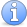## Techniques for maximizing and minimizing

### Quick description

Many important problems in mathematics concern the maximizing or minimizing of some quantity. This article contains links to articles about techniques for doing this.

### Extremal combinatorics front page

Extremal combinatorics is the branch of combinatorics devoted to finding combinatorial structures, such as graphs or subsets of the discrete cube, that maximize or minimize certain quantities subject to certain constraints.

### Variational methods front page

A typical example of a variational problem is to calculate the shape that a chain will form if its two ends are fixed. This is equivalent to minimizing the energy of the chain subject to certain constraints. The technique used to solve this problem is to find a local minimum by considering small variations to the position of the chain. However, this is more complicated than an elementary calculus problem because the space of possible positions of the chain is infinite dimensional.Incomplete This article is incomplete. This article is far from complete.